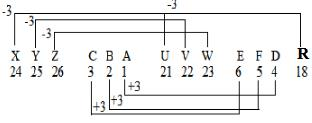Courses

# Consider the following statement: A simply supported beam is subjected to a couple somewhere in the span. It would produce.1. A rectangular SF diagram2. Parabolic BM diagrams3. both + ve and –ve BMswhich are maximum at the point of application of a couple of these statements-a)1, 2 and 3 are correctb)1 and 2 are correctc)2 and 3 are correctd)1 and 3 are correctCorrect answer is option 'D'. Can you explain this answer? Related Test: Mock Test: SSC JE Civil Engineering (CE)- 3

## SSC QuestionKnowledge Hub Jun 15, 2021∑Fy=0)
(RA⋅RB=0)
(∑MB=0)
(RAL−M=0)
(RA=M/L and RB=−(M/L))
Consider a section XX at a distance x from end B Shear force (SA−B=M/L)
Bending moment (Mx=Wx)
Hence the option D is correct.